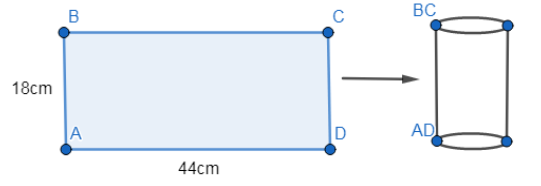QuestionAnswers

# A rectangular sheet of paper $44cm\times 18cm$ is rolled along its length and a cylinder is formed. The volume of the cylinder so formed is equal to (Take$\pi =\dfrac{22}{7}$ )A.2772cm3B.2506cm3C.2460cm3D.2672cm3Hint: Here, in this given question, when a rectangular sheet of paper $44cm\times 18cm$ is rolled along its length and a cylinder is formed, the width of the sheet becomes the height of the cylinder and the length becomes its base circumference. Then we just need to find the value of the radius of the base of the cylinder and put it in the formula for volume of a cylinder, $\pi {{r}^{2}}h$ and get our required answer.

In this given question, a rectangular sheet of paper $44cm\times 18cm$ is rolled along its length and a cylinder is formed and we are asked to find out the volume of the formed cylinder.
Now, when a rectangular sheet of paper $44cm\times 18cm$ is rolled along its length and a cylinder is formed, the width of the sheet becomes the height of the cylinder and the length becomes its base circumference as shown in the figure.So, the height of the cylinder is 18cm.
The base circumference is equal to 44cm.
This implies that,
\begin{align} & 2\pi r=44 \\ & \Rightarrow 2\times \dfrac{22}{7}\times r=44 \\ & \Rightarrow r=7cm \\ \end{align}
Now, the volume of a cylinder is equal to $\pi {{r}^{2}}h$.
So, putting the obtained value of r as 7cm in $\pi {{r}^{2}}h$, we get,
$volume=\pi {{r}^{2}}h=\dfrac{22}{7}\times {{7}^{2}}\times 18=2772c{{m}^{3}}$
Hence, we obtain the volume of the concerned cylinder as 2772cm3.
Therefore, we conclude that option (a) 2772cm3 is the correct answer to this given question.

Note: We should be careful to express the values in proper units. In this question as the length and breadth were given in terms of cm, the volume was obtained in terms of $c{{m}^{3}}$ , however, if the lengths were given in terms of other units such as meter, we should be careful to express the final answer by the corresponding units.
Where There is a Will There is a Way EssayIMO Sample Papers for Class 9Class 9 IMO Maths Olympiad Sample Question Paper - 1IMO Maths Olympiad Class 9 Previous Year Question PaperClass 9 IMO Maths Olympiad Sample Question Paper - 2IMO Maths Olympiad Class 9 - 2014 Previous Year Question PaperIMO Maths Olympiad Class 9 - 2013 Previous Year Question PaperIMO Maths Olympiad Class 9 - 2012 Previous Year Question PaperCBSE Date SheetCBSE Exam Date Sheet 2017 Class 12CBSE Exam Date Sheet 2020 Class 10CBSE Class 10 Date Sheet 2019CBSE Class 12 Date Sheet 2019CBSE Exam Date Sheet 2020 Class 12CBSE Exam Date Sheet 2018 Class 12Important Questions for CBSE Class 9 Maths Chapter 9 - Areas of Parallelograms and TrianglesImportant Questions for CBSE Class 9 Science Chapter 2 - Is Matter Around Us PureImportant Questions for CBSE Class 9 MathsCBSE Class 10 Hindi A Question Paper 2020Hindi A Class 10 CBSE Question Paper 2009Hindi A Class 10 CBSE Question Paper 2015Hindi A Class 10 CBSE Question Paper 2007Hindi A Class 10 CBSE Question Paper 2013Hindi A Class 10 CBSE Question Paper 2016Hindi A Class 10 CBSE Question Paper 2012Hindi A Class 10 CBSE Question Paper 2010Hindi A Class 10 CBSE Question Paper 2008Hindi A Class 10 CBSE Question Paper 2014NCERT Solutions for Class 9 English Moments Chapter 8 - A House is Not a HomeRD Sharma Class 9 Solutions Chapter 9 -Triangle and Its Angles (Ex 9.2) Exercise 9.2RD Sharma Class 9 Solutions Chapter 9 -Triangle and Its Angles (Ex 9.1) Exercise 9.1NCERT Solutions for Class 9 Maths Chapter 9 Areas of Parallelograms and Triangles (Ex 9.2) Exercise 9.2RD Sharma Class 11 Maths Solutions Chapter 22 - Brief Review of Cartesian System of Rectangular CoordinatesNCERT Solutions for Class 9 Maths Chapter 9 Areas of Parallelograms and Triangles (Ex 9.1) Exercise 9.1NCERT Solutions for Class 9 Maths Chapter 9 Areas of Parallelograms and Triangles (Ex 9.4) Exercise 9.4NCERT Solutions for Class 9 Maths Chapter 9 Areas of Parallelograms and Triangles (Ex 9.3) Exercise 9.3RS Aggarwal Class 9 Solutions Chapter-9 Congruence of Triangles and Inequalities in a TriangleNCERT Solutions for Class 9 Maths Chapter 9 Areas of Parallelograms and Triangles